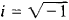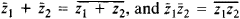# Conjugate Complex Numbers

The following article is from The Great Soviet Encyclopedia (1979). It might be outdated or ideologically biased.

## Conjugate Complex Numbers

two complex numbers of the form z = a + bi and z̄ = a- bi, where. Conjugate complex numbers are the roots of the quadratic equation

z2 – 2 az + a2 + b 2 = 0

with real coefficients. The sum and the product of two conjugate complex numbers are real. The conjugate of a sum is equal to the sum of the conjugates of the summands, and the conjugate of a product is equal to the product of the conjugates of the factors:. If z is a root of a polynomial with real coefficients, then z is also a root of the polynomial and is of the same multiplicity as z.